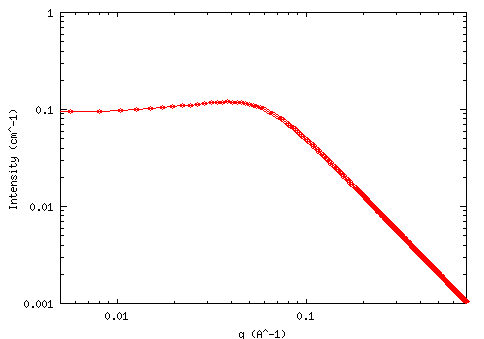MODEL

Borue-Erukhimovich RPA

AUTHOR/MODIFICATION

G. Nisato 30 NOV 1998

Alan Munter 08 JULY 1999, converted to Java

APPROVED FOR DISTRIBUTION

DESCRIPTION

Calculates the form factor, P(q), in the Borue-Erukhimovich random phase approximation (RPA) for a system of linear polyelectrolytes.

Resolution smeared version is also provided.

VARIABLES

Input Variables (default values):

Parameter Variable Value
0K (barns)10.0
1Lb (Å)7.1
2h (Å-3)12.0
3b (Å)10.0
4Cs (mol/L)0.0
5alpha0.05
6C (mol/L)0.7
7Incoherent Background (cm-1)0.0

USAGE NOTES

The function calculated is:

K = contrast factor of the polymer (barns = 10-24 cm2) = a2 with: a = (bp - vp/vsolvent * bsolvent)

where: bp = Sum of the scattering lengths of the atoms constituting the Monomer of the polymer under consideration

vp = partial molar volume of the polymer

vsolvent= partial molar volume of the solvent

Lb = Bjerrum length(Å)

This parameter needs to be kept constant for a given solvent and temperature!

h = virial parameter (Å3)

See Ref. (1) for the correct interpretation of this parameter. It incorporates second and third virial coefficients and can be negative.

b = monomer length (Å)

Cs = concentration of monovalent salt (mol/L)

Alpha = ionization degree : ratio of charged monomers to total number of monomers

C = polymer molar concentration (mol/L)

Bkd = Background

The returned value is in units of [cm-1]

REFERENCES

Borue, V. Y.; Erukhimovich, I. Y. Macromolecules 21, 3240 (1988).

Joanny, J.-F.; Leibler, L. Journal de Physique 51, 545 (1990).

Moussaid, A.; Schosseler, F.; Munch, J.-P.; Candau, S. J. Journal de Physique II France 3, 573 (1993).

Raphaël, E.; Joanny, J.-F. Europhysics Letters 11, 179 (1990).

TEST DATASET

This example dataset is produced by calculating the BE_RPA using 300 data points, qmin = 0.001 Å-1, qmax = 0.7 (Å-1) and the above default parameter values.